## Visualize Linear System of a Continuous-Time Model Discretized During Simulation

This example shows how to discretize a continuous-time model during simulation and plot the model's discretized linear behavior.

`scdcstr`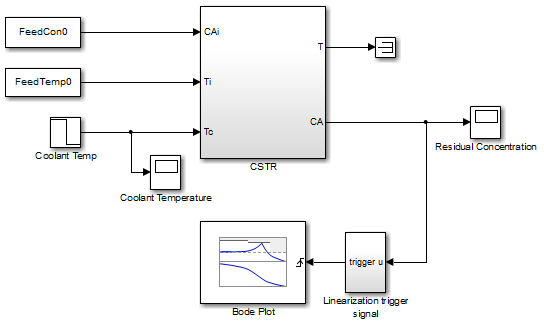In this model, the Bode Plot block has already been configured with:

• Input point at the coolant temperature input ```Coolant Temp```

• Output point at the residual concentration output `CA`

• Settings to linearize the model on a rising edge of an external trigger. The trigger signal is modeled in the ```Linearization trigger signal``` block in the model.

• Saving the computed linear system in the MATLAB® workspace as `LinearReactor`.

To view these configurations, double-click the block.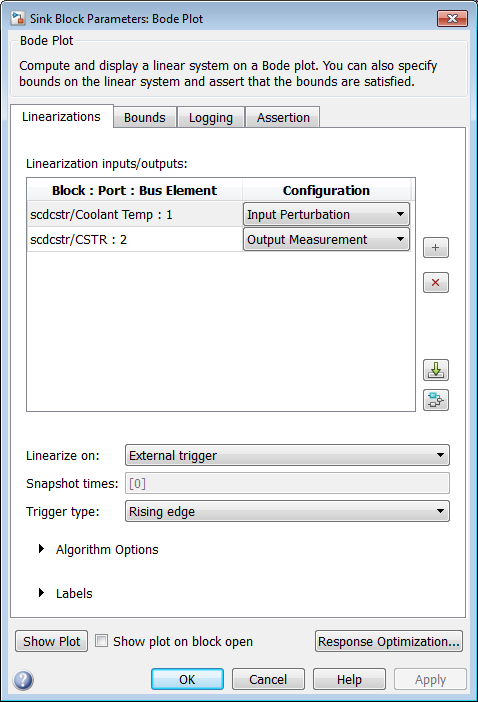2. Specify the sample time to compute the discrete-time linear system.

1. Clickadjacent to Algorithm Options.

The option expands to display the linearization algorithm options.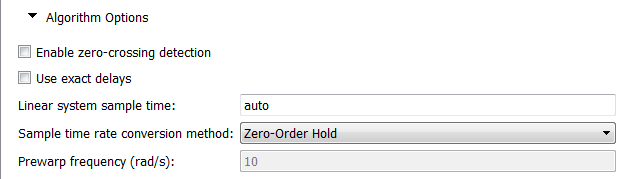2. Specify a sample time of 2 in the Linear system sample time field.

3. Click to open an empty Bode plot window.

4. Plot the Bode magnitude and phase by clicking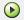in the plot window.

During simulation, the software:

• Linearizes the model on encountering a rising edge.

• Converts the continuous-time model into a discrete-time linear model with a sample time of 2. This conversion uses the default ```Zero-Order Hold``` method to perform the sample time conversion.

The software plots the discrete-time linear behavior in the Bode plot window. After the simulation completes, the plot window resembles the following figure.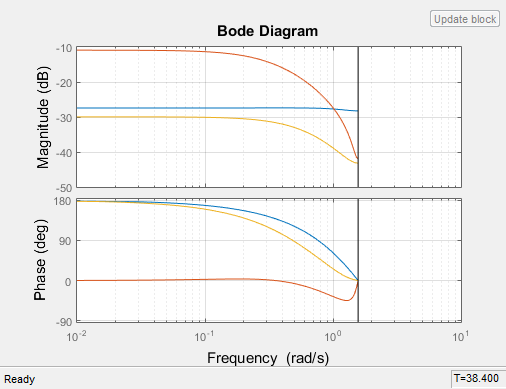The plot shows the Bode magnitude and phase up to the Nyquist frequency, which is computed using the specified sample time. The vertical line on the plot represents the Nyquist frequency.

## Related Topics